Example Questions

Example Question #1 : How To Find An Angle In A Right Triangle

In right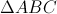,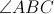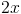and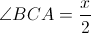.

What is the value of?

48

30

32

24

36

36

Explanation:

There are 180 degrees in every triangle. Since this triangle is a right triangle, one of the angles measures 90 degrees.

Therefore,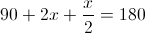.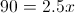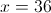Example Question #6 : Right Triangles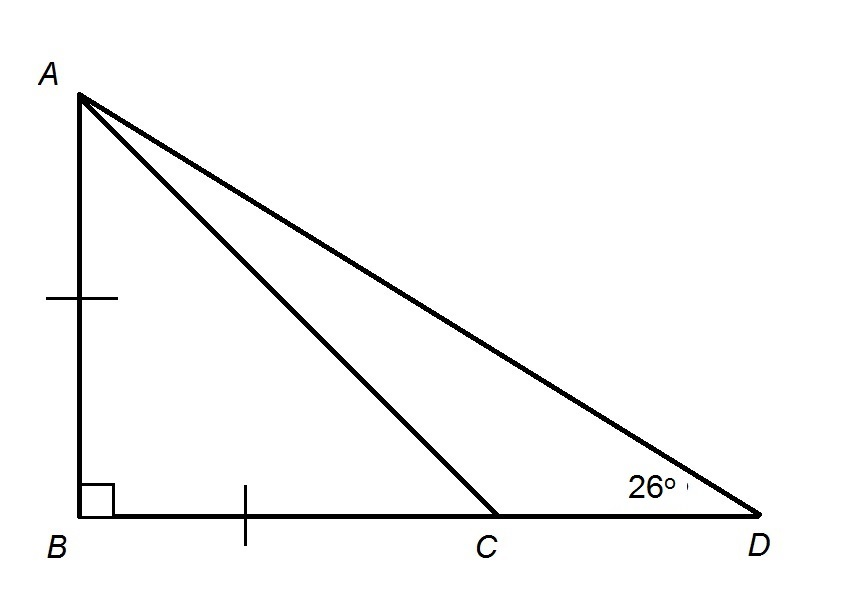Figure is not drawn to scale

Refer to the provided figure. Evaluate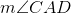.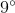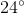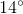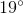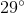Explanation: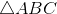is an isosceles right triangle with right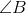, so, by the 45-45-90 Triangle Theorem,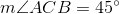. This angle is an exterior angle to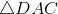,  so its measure is equal to the sum of those of its two remote interior angles,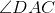and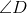. That is,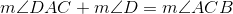Settingand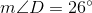, solve for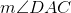: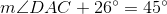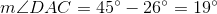Example Question #7 : Right TrianglesFigure not drawn to scale.

In the figure above, rays PA and PB are tangent to circle O at points A and B, respectively. If the diameter of circle O is 16 units and the length of line segment PO is 17 units, what is the area, in square units, of the quadrilateral PAOB?

68

120

60

240

136

120

Explanation:

Because PA and PB are tangent to circle O, angles PAO and PBO must be right angles; therefore, triangles PAO and PBO are both right triangles.

Since AO and OB are both radii of circle O, they are congruent. Furthermore, because PA and PB are external tangents originating from the same point, they must also be congruent.

So, in triangles PAO and PBO, we have two sides that are congruent, and we have a congruent angle (all right angles are congruent) between them. Therefore, by the Side-Angle-Side (SAS) Theorem of congruency, triangles PAO and PBO are congruent.

Notice that quadrilateral PAOB can be broken up into triangles PAO and PBO. Since those triangles are congruent, each must comprise one half of the area of quadrilateral PAOB. As a result, if we find the area of one of the triangles, we can double it in order to find the area of the quadrilateral.

Let's determine the area of triangle PAO. We have already established that it is a right triangle. We are told that PO, which is the hypotenuse of the triangle, is equal to 17. We are also told that the diameter of circle O is 16, which means that every radius of the circle is 8, because a radius is half the size of a diameter. Since segment AO is a radius, its length must be 8.

So, triangle PAO is a right triangle with a hypotenuse of 17 and a leg of 8. We can use the Pythagorean Theorem in order to find the other leg. According to the Pythagorean Theorem, if a and b are the lengths of the legs of a right triangle, and c is the length of the hypotenuse, then:

a2 + b2 = c2

Let us let b represent the length of PA.

82 + b2 = 172

64 + b2 = 289

Subtract 64 from both sides.

b2 = 225

Take the square root of both sides.

b = 15

This means that the length of PA is 15.

Now let's apply the formula for the area of a right triangle. Because the legs of a right triangle are perpendicular, one can be considered the base, and the other can be considered the height of the triangle.

area of triangle PAO = (1/2)bh

= (1/2)(8)(15) = 60

Ultimately, we must find the area of quadrilateral PAOB; however, we previously determined that triangles PAO and PBO each comprise half of the quadrilateral. Thus, if we double the area of PAO, we would get the area of quadrilateral PAOB.

Area of PAOB = 2(area of PAO)

= 2(60) = 120 square units

Example Question #8 : Right Triangles

If the hypotenuse of a triangle is 5 meters, which of the following is the closest value to the area of the triangle?

45

26

5

12

54

12

Explanation:

The answer is 12. In this circumstance, the area of the triangle cannot be smaller than its hypotenuse length, and cannot be bigger than its hypotenuse squared (that would be the area of a square).

Example Question #9 : Right Triangles

Triangle ABC is drawn between the points A(4, 3), B(4, 8), and C(7, 3). What is the area of ABC?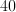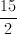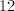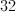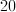Explanation:

Drawing a quick sketch of this triangle will reveal that it is a right triangle. The lines AB and AC form the height and base of this triangle interchangeably, depending on how you look at it.

Either way the formula for the area of the triangle is the distance from A to B multiplied by the distance from A to C, divided by 2.

This is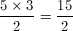Example Question #10 : Right Triangles

A right triangle has a total perimeter of 12, and the length of its hypotenuse is 5. What is the area of this triangle?

12

10

3

15

6

6

Explanation:

The area of a triangle is denoted by the equation 1/2 b x h.

b stands for the length of the base, and h stands for the height.

Here we are told that the perimeter (total length of all three sides) is 12, and the hypotenuse (the side that is neither the height nor the base) is 5 units long.

So, 12-5 = 7 for the total perimeter of the base and height.

7 does not divide cleanly by two, but it does break down into 3 and 4,

and 1/2 (3x4) yields 6.

Another way to solve this would be if you recall your rules for right triangles, one of the very basic ones is the 3,4,5 triangle, which is exactly what we have here

Example Question #6 : How To Find The Area Of A Right Triangle

The ratio for the side lengths of a right triangle is 3:4:5. If the perimeter is 48, what is the area of the triangle?

96

240

108

50

48

96

Explanation:

We can model the side lengths of the triangle as 3x, 4x, and 5x. We know that perimeter is 3x+4x+5x=48, which implies that x=4. This tells us that the legs of the right triangle are 3x=12 and 4x=16, therefore the area is A=1/2 bh=(1/2)(12)(16)=96.

Example Question #67 : Act Math

The length of one leg of an equilateral triangle is 6. What is the area of the triangle?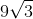Explanation: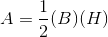The base is equal to 6.

The height of an quilateral triangle is equal to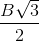, whereis the length of the base.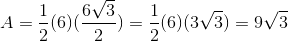Example Question #31 : Geometry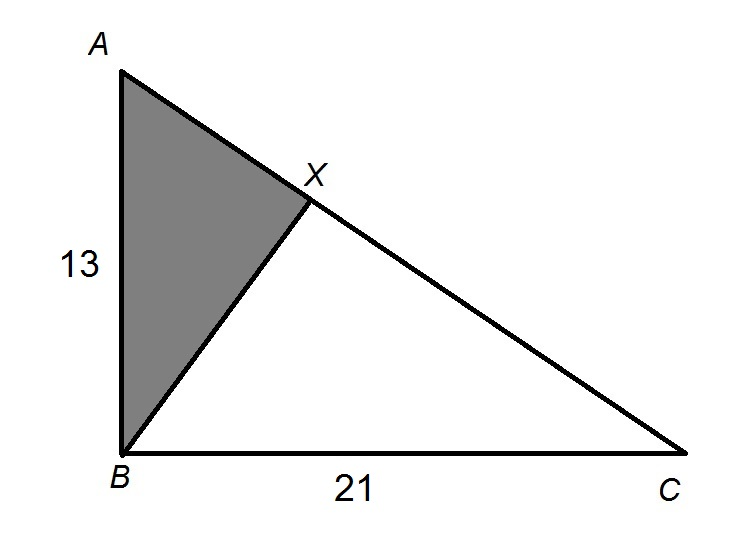Figure NOT drawn to scale.is a right triangle with altitude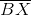. What percent ofis shaded in?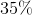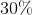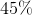Explanation:

The altitude of a right triangle from the vertex of its right angle - which, here, is- divides the triangle into two triangles similar to each other and to the large triangle. From the Pythagorean Theorem, the hypotenuse ofhas length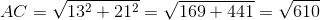.

The similarity ratio of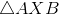tois the ratio of the lengths of the hypotenuses: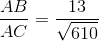The ratio of the areas of two similar triangles is the square of their similarity ratio, which here is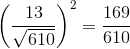Therefore, the area ofis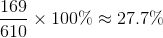the overall area of. This makesthe closest response.

Example Question #1 : How To Find The Area Of A Right Triangle

The perimeter of a right triangle is 40 units. If the lengths of the sides are,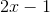, and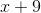units, then what is the area of the triangle?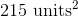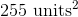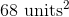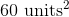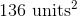Explanation:

Because the perimeter is equal to the sum of the lengths of the three sides of a triangle, we can add the three expressions for the lengths and set them equal to 40.

Perimeter: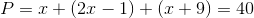Simplify the x terms.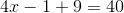Simplify the constants.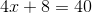Subtract 8 from both sides.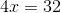Divide by 4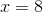One side is 8.

The second side is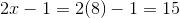.

The third side is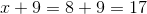.

Thus, the sides of the triangle are 8, 15, and 17.

The question asks us for the area of the triangle, which is given by the formula (1/2)bh. We are told it is a right triangle, so we can use one of the legs as the base, and the other leg as the height, since the legs will intersect at right angles. The legs of the right triangle must be the smallest sides (the longest must be the hypotenuse), which in this case are 8 and 15. So, let's assume that 8 is the base and 15 is the height.

The area of a triangle is (1/2)bh. We can substitute 8 and 15 for b and h.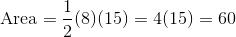.

The answer is 60 units squared.